# User:Vishnu

My GSoC 2016 project will be on the topic Adding incompressible fluids EOS to FPROPS. The project details and proposal are on the official GSOC website. My mentors will be John Pye and Mahesh Venkataraman.

My code is at vishnu:.

# Approach

Here are some examples of current incompressible fluid support in ASCEND:

The project is divided into two parts:

1. Develop a stand alone code for computing popular thermodynamic properties for incompressible pure fluids as well as mixtures.
• This includes development of new evaluation functions for incompressible fluids based on polynomial, exponential and exponential-polynomial mathematical models with fitting coefficients extracted from various published journal articles. The development of the stand alone code will finally consist of extensive verification and validation with available data in CoolProp. The deadline to complete this work will be June 27, 2016 (GSoC '16 mid-term).
2. Enhance the FRPOPS module with the features of the developed stand alone code
• This second part (post mid-term) will consist of developing the incompressible fluid data structures from the existing structures in FPROPS. The property evaluation functions developed in the first part will be then linked with FPROPS, hence completely adding the incompressible EOS to FPROPS.

## Before 23 May 2016 (community bonding period and before)

1. Active participation with ascend-sim-users link
2. Executed several ready made ASCEND models (for nitrogen and oxygen) provided by Dr. John Pye (that invoke FPROPS)
3. Understood the implementation of TTSE (done by Dr. Sidharth Somanathan, GSoC '15 student) link
4. Reported minor bug related to the test for 'Nitrogen' link

Work under progress:

1. Understand call graph and data flow of ASCEND-FPROPS models

## 30 May 2016

1. Identified existing incompressible models written for a pure fluid (liquidsodium) and a molten mixture (KNO3:NaNO3)
2. Literature review
• Found repositories (in JSON format from CoolProp) for extracting coefficient matrices (as discussed in the proposal) of incompressible fluid models (above 100 pure and mixture fluids)
• Different evaluation functions required for different types of fit coefficients are: polynomial type, exponential type and exponential-polynomial type

Work under progress:

1. Understand the implementation of incompressible fluid models in CoolProp
• Extract the coefficient matrix by implementing a JSON-to-C file parser
• Implement the evaluation functions

## 06 June 2016

1. Identified relevant 'C' structures required for incompressible fluids

```typedef struct {
char* type;
double** coeff;
int numc_r, numc_c;	// number of rows and columns in coefficient matrix respectively
} coefficients;

typedef struct {
coefficients T_freeze, conductivity, density, specific_heat, viscosity, saturation_pressure;
char* x_id;
char* description;
double T_base, T_max, T_min, T_minPsat, x_base, x_max, x_min;
} fprops_incompressible;
```
1. JSON-to-C file parser added to the standalone code and subsequently loaded the incompressible fluid data structures

Work under progress:

1. Adding property evaluation functions to the standalone code
• for 'density', 'conductivity', 'heat capacity' and 'viscosity'
• as function of 'temperature' (as discussed in the proposal)

## 13 June 2016

1. Still working on incompressible standalone code (please review the committed work)
2. Added property evaluation functions for density, conductivity, heat capacity and viscosity
3. Added functions to compute properties from different types of fit coefficients: polynomial, exponential, exponential-polynomial
4. Analyzed preliminary results for "Zitrec S55, Potassium formate/Sodium propionate"

Work under progress:

1. Comparing with respective computations of CoolProp to detect the root-cause behind mismatching results

Future discussion:

1. What is the purpose of "NRMS" (normalized root-mean-square provided in the 'JSON' files of CoolProp)? Do I have to include that anywhere in the calculations or is it just additional info?
NRMS is just a measure of correlation/agreement between results. When the value is low, it means there is good agreement. Note the ambiguity in the definition around 'nomalized'

## 20 June 2016

1. Dived deep into CoolProp evaluation functions and debugged the existing code for 'pure' fluids.
2. Ran test cases for
• Zitrec S55, Potassium Formate/Sodium Propionate (to test polynomial and exp-polynomial functions) and
• Water (exponential viscosity model)
3. Exponential type model for viscosity - bugs:
• The model was not based on a centered-fit approach.
• There is no need to subtract the base property (Temperature in our case) before evaluating the model.
• Also, there is a change in sign for one of the viscosity coefficients entered in the original JSON files.
4. Demonstrated exact results to that generated by CoolProp (compare Original_*.pdf to Results_*.pdf in repository)

Conclusion: Pure fluids incompressible EOS ready to be merged with FPROPS

Work under progress till July 27 (GSoC mid-term):

1. Get the EOS for incompressible mixtures working: Demonstrate match for LiBr
2. Add integration functions to evaluate 'internal energy','enthalpy' and 'entropy' (as discussed in proposal)

Further Discussions:

1. Please note that models for incompressible mixtures are further complicated as we now have 2D coefficient matrices. Are there simplified models?

## 27 June 2016 (GSoC '16 mid-term)

Here is the update:

1. Developed a working stand alone code for evaluating incompressible EOS (pure fluids and mixtures)
• Consists of JSON-to-C parser (for current requirements)
• Evaluates different types of incompressible EOS: polynomial, exponential-polynomial and exponential for pure and mixed fluids
• Evaluation functions for different major thermodynamic properties: Density, conductivity, specific heat, viscosity, saturation pressure
2. Performed test cases for
• Zitrec S55, Potassium formate/Sodium propionate (to test polynomial and exp-polynomial functions)
• Water (exponential viscosity model) and
• LiBr (mixture)
• Demonstrated exact results to those generated by CoolProp (compare Original_*.pdf to Results_*.pdf in the repository) [* = ZS55, Water, LiBr]
Verification and validation of incompressible EOS models for pure fluid (Water) and mixture (Lithium Bromide)
Original from CoolProp Test results Test results Test results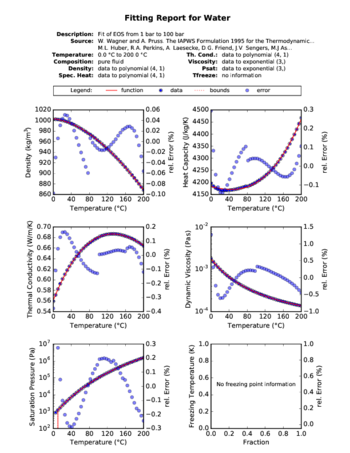'Density' (polynomial fit), 'Heat Capacity' (polynomial fit), 'Thermal Conductivity' (polynomial fit), 'Dynamic Viscosity' (exponential fit) and 'Saturation Pressure' (exponential fit) profiles of Water (pure fluid)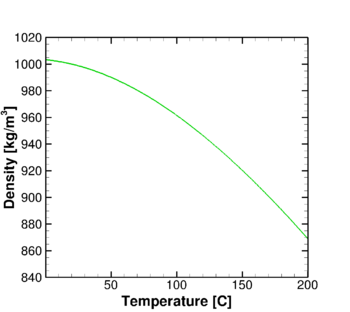Polynomial fit of 'Density' profile for Water (pure fluid) mixture generated by the standalone code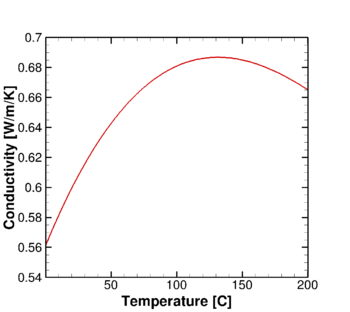Polynomial fit of 'Thermal Conductivity' profile for Water (pure fluid) mixture generated by the standalone code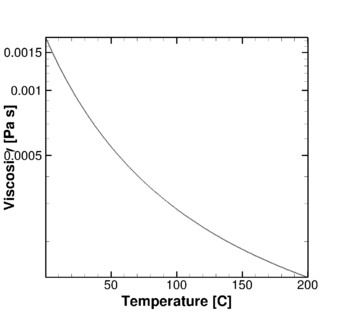Exponential fit of 'Dynamic Viscosity' profile for Water (pure fluid) mixture generated by the standalone code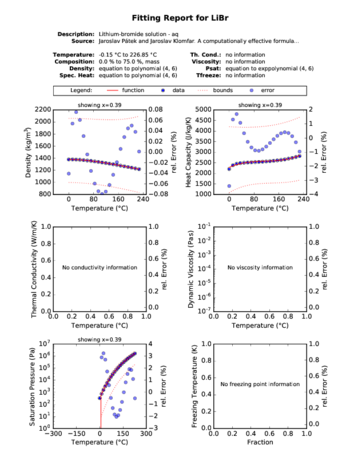'Density' (polynomial fit), 'Heat Capacity' (polynomial fit) and 'Saturation Pressure' (exponential-polynomial fit) profiles of LiBr mixture at x = 0.39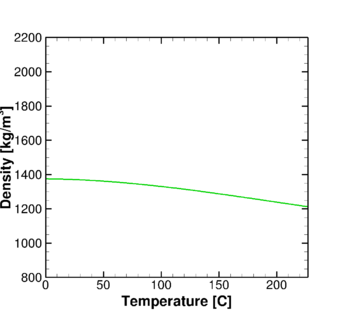Polynomial fit of 'Density' profile for LiBr mixture generated by the standalone code at x = 0.39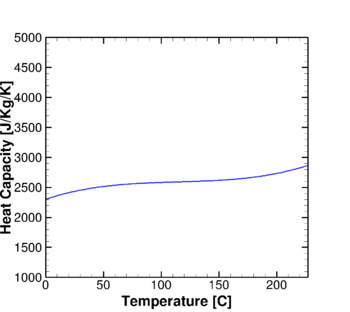Polynomial fit of 'Heat Capacity' profile for LiBr mixture generated by the standalone code at x = 0.39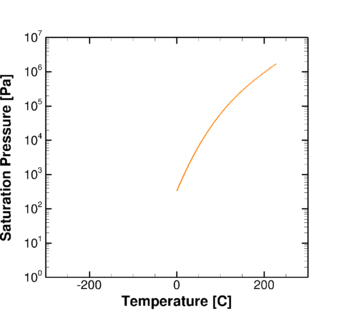Exponential-polynomial fit of 'Saturation Pressure' profile for LiBr mixture generated by the standalone code at x = 0.39

Conclusion: Pure and mixed fluids incompressible EOS ready to be linked with FPROPS

Work under progress:

1. Add integration functions to evaluate internal energy/enthalpy/entropy
2. Start final integration of the current code with FPROPS

Further Discussions:

1. Do you know of any published data/equations/results to compare the integrated properties (internal energy/enthalpy/entropy)?

## 03 July 2016

• It is assumed that specific heats are polynomial functions of input temperature
• Integration functions for internal energy and entropy calculations for incompressible pure fluid and mixtures are then added according to the equations given in the proposal
• All incompressible fluids have an arbitrary reference state for internal energy and entropy: During initialization, the reference state is defined as a temperature of 20 C and a pressure of 1 atm according to the U.S. National Institute of Standards and Technology.

Further discussions:

1. We don't know if we need a centered fit for this computation too (as in the evaluation functions before)
• No data available for validation purposes
2. How do we deal with enthalpy computation?
• This depends on input temperature as well as pressure

Work under progress:

1. Adding incompressible fluid data structures to filedata.h and rundata.h

## 10 July 2016

1. Modified the files filedata.h and rundata.h to store incompressibile fluid coefficients
• Note that viscosity and thermal conductivity coefficients for incompressible fluids are bound with the incompressible fluid data structure (although there is an option to separate them out)
• New pointers to departure functions added into PureFluid_struct to facilitate computations of some incompressible fluid properties (freezing temperature, viscosity, saturation pressure etc.)

Work under progress:

1. Adding files incompressible.h and incompressible.c
• Most of the evaluation function will have to be simply copied from the working/validated standalone code

## 17 July 2016

1. rundata.h and filedata.h in good shape
• compatible with both pure and mixture incompresible fluids
2. Evaluation function declaration and definition completed in incompressible.h and incompressible.c file respectively (except enthalpy, internal energy and entropy)

Work under progress:

1. Write the incompressible_prepare() subroutine to load the stored model data from filedata to rundata
2. Completing evaluation function for integrated properties (enthalpy, internal energy and entropy)
3. Learn final linking of incompressible model through fprops(.h/.c) files

Further discussions:

1. Which are the files that need major changes while performing the final binding work (of the incompressible model to FPROPS)?

## 24 July 2016

1. Finished coding up the evaluation function for integrated properties (internal energy and entropy)
2. Completed the incompressible_prepare() subroutine: link

Under progress:

1. Binding the incompressible model with FPROPS
2. Setting up test cases similar to what was done with the standalone code
• Focus on Zitrec S55, Potassium Formate/Sodium Propionate (pure) and LiBr (mixture) verification

## 31 July 2016

1. Initial binding of the incompressible model to FPROPS successful
2. Incompressible module tested for Zitrec S55, Potassium Formate/Sodium Propionate (pure) and LiBr (mixture) solution
• Results consistent with previous test performed with the standalone code
• Please find the relevant input, source code, post-processing .py scripts and output+plots here: (moved to) link1 and link2

Further discussion:

1. Here, the underlying fluid state structure in 'fprops' file had to be changed to incorporate the values for concentration (x) and pressure (p)
• This might affect the assumption made by other EOS models in FPROPS

Work under progress:

1. Concentrate binding the pure incompressible fluid part
• This can be done without changing the fluid state structure
• But then, it should be noted that it will be 'pressure' that will be actually stored in the variable named 'density' during incompressible EOS computations
• The mixture part that was coded up will be placed in a backup folder and will be worked upon in the future (post-GSoC '16)

## 07 August 2016

1. fprops.h and fprops.c files edited to support pure incompressible EOS
2. Final binding of 'pure' incompressible EOS model completed

Work under progress:

1. Verification of incompressible EOS model with available ascend-model for 'molten salt'

## 14 August 2016

1. Managed to partially get the test case for molten-salt working
• Approach was to create a 'json' file for the coefficients as given in http://prod.sandia.gov/techlib/access-control.cgi/2001/012100.pdf
• Debugging and tedious unit conversions (from British to SI and back)
• Deviations from the SNL paper data are significant for density and specific heat
• Here are the values (original data, fitted data from code) all in SI units at (T,p) = (1050 F, 1 bar):
• density (1728,1730)
• specific heat (1544,1540)
• viscosity (1.14e-3,1.14e-3)
• conductivity (0.55,0.55)

Further work:

1. Finish molten-salt test case with appropriate error analysis

Discussion:

1. No validation data to compare against for integrated properties.
2. How to deal with enthalpy calculation? (pressure comes into picture)
3. Discussion about functions on computing the reference values: Is it really necessary to add reference states in the evaluation functions if there are many options (and since we don't know which one to use for incompressible fluids)? I assume that the CFD solvers are more concerned with the gradients rather than the absolute values. Or is it important when it comes to computational chemistry?

## 21 August 2016 (GSoC '16 Final Product)

2. The test case presented will be that for 'molten salt'

How to run the test in your Linux machine (assuming svn, scons and other relevant apps are already installed):

• Check out the code from my repository using svn client:

```svn co http://svn.ascend4.org/branches/vishnu ascend
```
• Run scons script from fprops folder:

```cd ascend/models/johnpye/fprops
```

```scons
```
• Run the moltensalt_btu_test executable in the test folder:

```cd test // or cd ascend/models/johnpye/fprops/test
```

```./moltensalt_btu_test
```

Output:

```incompressible.c:107(incompressible_prepare): Incompressible

Test point: 0 {Temperature 	Density 	Specific Heat 	Viscosity 	Conductivity}
FPROPS at T = 5.000000e+02	1.200950e+02	3.564000e-01	1.050580e+01	2.845572e-01
Error at T =  5.000000e+02	0.000000e+00	0.000000e+00	0.000000e+00	0.000000e+00

Test point: 1 {Temperature 	Density 	Specific Heat 	Viscosity 	Conductivity}
FPROPS at T = 5.500000e+02	1.189845e+02	3.575400e-01	8.607339e+00	2.876921e-01
Error at T =  5.500000e+02	0.000000e+00	0.000000e+00	0.000000e+00	0.000000e+00

Test point: 2 {Temperature 	Density 	Specific Heat 	Viscosity 	Conductivity}
FPROPS at T = 6.000000e+02	1.178740e+02	3.586800e-01	7.085347e+00	2.908270e-01
Error at T =  6.000000e+02	0.000000e+00	0.000000e+00	0.000000e+00	0.000000e+00

Test point: 3 {Temperature 	Density 	Specific Heat 	Viscosity 	Conductivity}
FPROPS at T = 6.500000e+02	1.167635e+02	3.598200e-01	5.893968e+00	2.939620e-01
Error at T =  6.500000e+02	0.000000e+00	0.000000e+00	0.000000e+00	0.000000e+00

Test point: 4 {Temperature 	Density 	Specific Heat 	Viscosity 	Conductivity}
FPROPS at T = 7.000000e+02	1.156530e+02	3.609600e-01	4.987346e+00	2.970969e-01
Error at T =  7.000000e+02	0.000000e+00	0.000000e+00	0.000000e+00	0.000000e+00

Test point: 5 {Temperature 	Density 	Specific Heat 	Viscosity 	Conductivity}
FPROPS at T = 7.500000e+02	1.145425e+02	3.621000e-01	4.319625e+00	3.002318e-01
Error at T =  7.500000e+02	0.000000e+00	0.000000e+00	0.000000e+00	0.000000e+00

Test point: 6 {Temperature 	Density 	Specific Heat 	Viscosity 	Conductivity}
FPROPS at T = 8.000000e+02	1.134320e+02	3.632400e-01	3.844950e+00	3.033667e-01
Error at T =  8.000000e+02	0.000000e+00	0.000000e+00	0.000000e+00	0.000000e+00

Test point: 7 {Temperature 	Density 	Specific Heat 	Viscosity 	Conductivity}
FPROPS at T = 8.500000e+02	1.123215e+02	3.643800e-01	3.517466e+00	3.065016e-01
Error at T =  8.500000e+02	0.000000e+00	0.000000e+00	0.000000e+00	0.000000e+00

Test point: 8 {Temperature 	Density 	Specific Heat 	Viscosity 	Conductivity}
FPROPS at T = 9.000000e+02	1.112110e+02	3.655200e-01	3.291317e+00	3.096366e-01
Error at T =  9.000000e+02	0.000000e+00	0.000000e+00	0.000000e+00	0.000000e+00

Test point: 9 {Temperature 	Density 	Specific Heat 	Viscosity 	Conductivity}
FPROPS at T = 9.500000e+02	1.101005e+02	3.666600e-01	3.120647e+00	3.127715e-01
Error at T =  9.500000e+02	0.000000e+00	0.000000e+00	0.000000e+00	0.000000e+00

Test point: 10 {Temperature 	Density 	Specific Heat 	Viscosity 	Conductivity}
FPROPS at T = 1.000000e+03	1.089900e+02	3.678000e-01	2.959600e+00	3.159064e-01
Error at T =  1.000000e+03	0.000000e+00	0.000000e+00	0.000000e+00	0.000000e+00

Test point: 11 {Temperature 	Density 	Specific Heat 	Viscosity 	Conductivity}
FPROPS at T = 1.050000e+03	1.078795e+02	3.689400e-01	2.762321e+00	3.190413e-01
Error at T =  1.050000e+03	0.000000e+00	0.000000e+00	0.000000e+00	0.000000e+00

Test point: 12 {Temperature 	Density 	Specific Heat 	Viscosity 	Conductivity}
FPROPS at T = 1.100000e+03	1.067690e+02	3.700800e-01	2.482955e+00	3.221762e-01
Error at T =  1.100000e+03	0.000000e+00	0.000000e+00	0.000000e+00	0.000000e+00
```